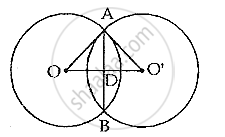Share

The Length of Common Chord of Two Intersecting Circles is 30 Cm. If the Diameters of These Two Circles Be 50 Cm and 34 Cm, Calculate the Distance Between Their Centres. - ICSE Class 10 - Mathematics

ConceptChord Properties - a Straight Line Drawn from the Center of a Circle to Bisect a Chord Which is Not a Diameter is at Right Angles to the Chord

Question

The length of common chord of two intersecting circles is 30 cm. If the diameters of these two circles be 50 cm and 34 cm, calculate the distance between their centres.

SolutionOA = 25 cm and AB = 30 cm

∴ AD = 1/2× AB =(1/2×30)cm = 15 cm

Now in right angled ΔADO,

OA^2 = AD^2 + OD^2
⇒ OD^2 = OA^2 - OD^2 = 25^2 -15^2
= 625 - 225 = 400

∴ OD =sqrt 400 = 20 cm
Again, we have O 'A = 17 cm
In right angle ΔADO'
 O'A ^2= AD^2 +O'D^2
⇒ O'D^2 =O'A^2 - AD^2 = 17^2 -15^2
⇒ 289 - 225 = 64
∴ O'D 8 cm
∴ OO' (OD+ O'D)
= (20 +8)= 28 cm
∴ the distance between their centres is 28 cm

Is there an error in this question or solution?

Video TutorialsVIEW ALL 

Solution The Length of Common Chord of Two Intersecting Circles is 30 Cm. If the Diameters of These Two Circles Be 50 Cm and 34 Cm, Calculate the Distance Between Their Centres. Concept: Chord Properties - a Straight Line Drawn from the Center of a Circle to Bisect a Chord Which is Not a Diameter is at Right Angles to the Chord.
S